x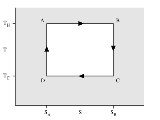Carnot cycleOverview

The Carnot cycle is a theoretical thermodynamic cycle
Thermodynamic cycle
A thermodynamic cycle consists of a series of thermodynamic processes transferring heat and work, while varying pressure, temperature, and other state variables, eventually returning a system to its initial state...

proposed by Nicolas Léonard Sadi Carnot
Nicolas Léonard Sadi Carnot
Nicolas Léonard Sadi Carnot was a French military engineer who, in his 1824 Reflections on the Motive Power of Fire, gave the first successful theoretical account of heat engines, now known as the Carnot cycle, thereby laying the foundations of the second law of thermodynamics...

in 1824 and expanded by Benoit Paul Émile Clapeyron in the 1830s and 40s. It can be shown that it is the most efficient cycle for converting a given amount of thermal energy into work, or conversely, creating a temperature difference (e.g. refrigeration) by doing a given amount of work.

Every thermodynamic system
Thermodynamic system
A thermodynamic system is a precisely defined macroscopic region of the universe, often called a physical system, that is studied using the principles of thermodynamics....

exists in a particular thermodynamic state
Thermodynamic state
A thermodynamic state is a set of values of properties of a thermodynamic system that must be specified to reproduce the system. The individual parameters are known as state variables, state parameters or thermodynamic variables. Once a sufficient set of thermodynamic variables have been...

.Unanswered QuestionsHow many processes are in the carnot cycleWhy carnot cycle take two isothermal and two isentropic process for highest effiency. Constant pressure heat addition will give more work. Is it co...carnot efficiencyDiscussionsWhat is carnot cycle?carnot efficiencyEncyclopedia
The Carnot cycle is a theoretical thermodynamic cycle
Thermodynamic cycle
A thermodynamic cycle consists of a series of thermodynamic processes transferring heat and work, while varying pressure, temperature, and other state variables, eventually returning a system to its initial state...

proposed by Nicolas Léonard Sadi Carnot
Nicolas Léonard Sadi Carnot
Nicolas Léonard Sadi Carnot was a French military engineer who, in his 1824 Reflections on the Motive Power of Fire, gave the first successful theoretical account of heat engines, now known as the Carnot cycle, thereby laying the foundations of the second law of thermodynamics...

in 1824 and expanded by Benoit Paul Émile Clapeyron in the 1830s and 40s. It can be shown that it is the most efficient cycle for converting a given amount of thermal energy into work, or conversely, creating a temperature difference (e.g. refrigeration) by doing a given amount of work.

Every thermodynamic system
Thermodynamic system
A thermodynamic system is a precisely defined macroscopic region of the universe, often called a physical system, that is studied using the principles of thermodynamics....

exists in a particular thermodynamic state
Thermodynamic state
A thermodynamic state is a set of values of properties of a thermodynamic system that must be specified to reproduce the system. The individual parameters are known as state variables, state parameters or thermodynamic variables. Once a sufficient set of thermodynamic variables have been...

. When a system is taken through a series of different states and finally returned to its initial state, a thermodynamic cycle is said to have occurred. In the process of going through this cycle, the system may perform work on its surroundings, thereby acting as a heat engine
Heat engine
In thermodynamics, a heat engine is a system that performs the conversion of heat or thermal energy to mechanical work. It does this by bringing a working substance from a high temperature state to a lower temperature state. A heat "source" generates thermal energy that brings the working substance...

. A system undergoing a Carnot cycle is called a Carnot heat engine
Carnot heat engine
A Carnot heat engine is a hypothetical engine that operates on the reversible Carnot cycle. The basic model for this engine was developed by Nicolas Léonard Sadi Carnot in 1824...

, although such a 'perfect' engine is only a theoretical limit and cannot be built in practice.

The Carnot cycle when acting as a heat engine consists of the following steps:
1. Reversible isothermal expansion of the gas at the "hot" temperature, TH (isothermal heat addition). During this step (A to B on Figure 1, 1 to 2 in Figure 2) the expanding gas makes the piston work on the surroundings. The gas expansion is propelled by absorption of quantity Q1 of heat from the high temperature reservoir.
2. Isentropic
Isentropic process
In thermodynamics, an isentropic process or isoentropic process is one in which for purposes of engineering analysis and calculation, one may assume that the process takes place from initiation to completion without an increase or decrease in the entropy of the system, i.e., the entropy of the...

(reversible adiabatic) expansion of the gas (isentropic work output).
For this step (B to C on Figure 1, 2 to 3 in Figure 2) the piston and cylinder are assumed to be thermally insulated, thus they neither gain nor lose heat. The gas continues to expand, working on the surroundings. The gas expansion causes it to cool to the "cold" temperature, TC.
3. Reversible isothermal compression of the gas at the "cold" temperature, TC. (isothermal heat rejection) (C to D on Figure 1, 3 to 4 on Figure 2) Now the surroundings do work on the gas, causing quantity Q2 of heat to flow out of the gas to the low temperature reservoir.
4. Isentropic compression of the gas (isentropic work input). (D to A on Figure 1, 4 to 1 in Figure 2) Once again the piston and cylinder are assumed to be thermally insulated. During this step, the surroundings do work on the gas, compressing it and causing the temperature to rise to TH. At this point the gas is in the same state as at the start of step 1.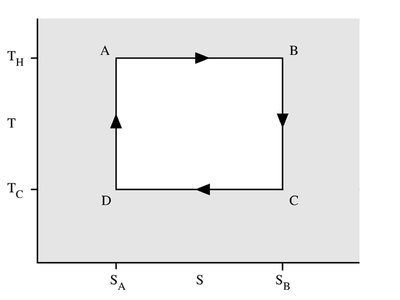### The temperature-entropy diagram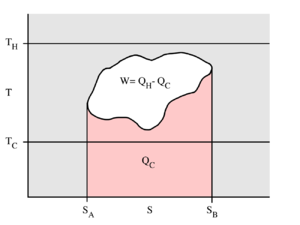The behaviour of a Carnot engine or refrigerator is best understood by using a temperature-entropy (TS) diagram, in which the thermodynamic state is specified by a point on a graph with entropy
Entropy
Entropy is a thermodynamic property that can be used to determine the energy available for useful work in a thermodynamic process, such as in energy conversion devices, engines, or machines. Such devices can only be driven by convertible energy, and have a theoretical maximum efficiency when...

(S) as the horizontal axis and temperature (T) as the vertical axis. For a simple system with a fixed number of particles, any point on the graph will represent a particular state of the system. A thermodynamic process will consist of a curve connecting an initial state (A) and a final state (B). The area under the curve will be: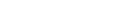which is the amount of thermal energy transferred in the process. If the process moves to greater entropy, the area under the curve will be the amount of heat absorbed by the system in that process. If the process moves towards lesser entropy, it will be the amount of heat removed. For any cyclic process, there will be an upper portion of the cycle and a lower portion. For a clockwise cycle, the area under the upper portion will be the thermal energy absorbed during the cycle, while the area under the lower portion will be the thermal energy removed during the cycle. The area inside the cycle will then be the difference between the two, but since the internal energy of the system must have returned to its initial value, this difference must be the amount of work done by the system over the cycle. Referring to figure 2, mathematically, for a reversible process we may write the amount of work done over a cyclic process as: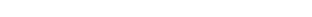Since dU is an exact differential
Exact differential
A mathematical differential is said to be exact, as contrasted with an inexact differential, if it is of the form dQ, for some differentiable function Q....

, its integral over any closed loop is zero and it follows that the area inside the loop on a T-S diagram is equal to the total work performed if the loop is traversed in a clockwise direction, and is equal to the total work done on the system as the loop is traversed in a counterclockwise direction.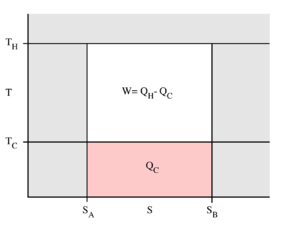### The Carnot cycle

Evaluation of the above integral is particularly simple for the Carnot cycle. The amount of energy transferred as work is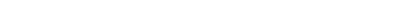The total amount of thermal energy transferred between the hot reservoir and the system will be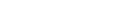and the total amount of thermal energy transferred between the system and the cold reservoir will be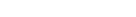The efficiency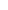is defined to be: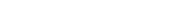where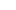is the work done by the system (energy exiting the system as work),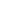is the heat put into the system (heat energy entering the system),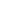is the absolute temperature of the cold reservoir, and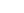is the absolute temperature of the hot reservoir.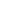is the maximum system entropy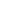is the minimum system entropy

This efficiency makes sense for a heat engine
Heat engine
In thermodynamics, a heat engine is a system that performs the conversion of heat or thermal energy to mechanical work. It does this by bringing a working substance from a high temperature state to a lower temperature state. A heat "source" generates thermal energy that brings the working substance...

, since it is the fraction of the heat energy extracted from the hot reservoir and converted to mechanical work. A Rankine cycle
Rankine cycle
The Rankine cycle is a cycle that converts heat into work. The heat is supplied externally to a closed loop, which usually uses water. This cycle generates about 90% of all electric power used throughout the world, including virtually all solar thermal, biomass, coal and nuclear power plants. It is...

is usually the practical approximation.

### Carnot's theorem

It can be seen from the above diagram, that for any cycle operating between temperaturesand, none can exceed the efficiency of a Carnot cycle.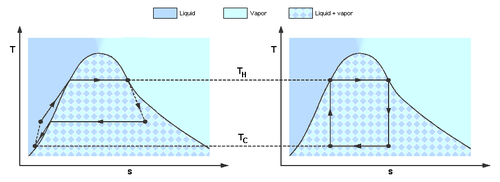Carnot's theorem is a formal statement of this fact: No engine operating between two heat reservoirs can be more efficient than a Carnot engine operating between those same reservoirs. Thus, Equation 3 gives the maximum efficiency possible for any engine using the corresponding temperatures. A corollary to Carnot's theorem states that: All reversible engines operating between the same heat reservoirs are equally efficient. Rearranging the right side of the equation gives what may be a more easily understood form of the equation. Namely that the theoretical maximum efficiency of a heat engine equals the difference in temperature between the hot and cold reservoir divided by the absolute temperature of the hot reservoir. To find the absolute temperature in kelvin, add 273.15 degrees to the Celsius temperature. Looking at this formula an interesting fact becomes apparent. Lowering the temperature of the cold reservoir will have more effect on the ceiling efficiency of a heat engine than raising the temperature of the hot reservoir by the same amount. In the real world, this may be difficult to achieve since the cold reservoir is often an existing ambient temperature.

In other words, maximum efficiency is achieved if and only if no new entropy is created in the cycle. Otherwise, since entropy is a state function
State function
In thermodynamics, a state function, function of state, state quantity, or state variable is a property of a system that depends only on the current state of the system, not on the way in which the system acquired that state . A state function describes the equilibrium state of a system...

, the required dumping of heat into the environment to dispose of excess entropy leads to a reduction in efficiency. So Equation 3 gives the efficiency of any reversible
Reversible process (thermodynamics)
In thermodynamics, a reversible process, or reversible cycle if the process is cyclic, is a process that can be "reversed" by means of infinitesimal changes in some property of the system without loss or dissipation of energy. Due to these infinitesimal changes, the system is in thermodynamic...

heat engine
Heat engine
In thermodynamics, a heat engine is a system that performs the conversion of heat or thermal energy to mechanical work. It does this by bringing a working substance from a high temperature state to a lower temperature state. A heat "source" generates thermal energy that brings the working substance...

.

In mesoscopic heat engines, work per cycle of operation fluctuates due to thermal noise.
For the case when work and heat fluctuations are counted, there is exact equality that relates average of exponents of work performed by any heat engine and the heat transfer from the hotter heat bath . This relation transforms the Carnot's inequality into exact equality that is applied to an arbitrary heat engine coupled to two heat reservoirs and operating at arbitrary rate.

### Efficiency of real heat engines

Carnot realized that in reality it is not possible to build a thermodynamically reversible engine, so real heat engines are less efficient than indicated by Equation 3. Nevertheless, Equation 3 is extremely useful for determining the maximum efficiency that could ever be expected for a given set of thermal reservoirs.

Although Carnot's cycle is an idealisation, the expression
of Carnot efficiency is still useful. Consider the average
Average
In mathematics, an average, or central tendency of a data set is a measure of the "middle" value of the data set. Average is one form of central tendency. Not all central tendencies should be considered definitions of average....

temperatures,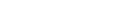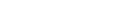at which heat is input and output, respectively. Replace TH and TC in Equation (3) by <TH> and <TC> respectively.

For the Carnot cycle, or its equivalent, <TH> is the highest temperature available and <TC> the lowest. For other less efficient cycles, <TH> will be lower than TH , and <TC> will be higher than TC. This can help illustrate, for example, why a reheater
Economizer
Economizers , or economisers , are mechanical devices intended to reduce energy consumption, or to perform another useful function such as preheating a fluid. The term economizer is used for other purposes as well. Boiler, powerplant, and heating, ventilating, and air-conditioning uses are...

or a regenerator can improve thermal efficiency.
See also: Heat Engine efficiency and other performance criteria

## See also

• Carnot heat engine
Carnot heat engine
A Carnot heat engine is a hypothetical engine that operates on the reversible Carnot cycle. The basic model for this engine was developed by Nicolas Léonard Sadi Carnot in 1824...

• Reversible process (thermodynamics)
Reversible process (thermodynamics)
In thermodynamics, a reversible process, or reversible cycle if the process is cyclic, is a process that can be "reversed" by means of infinitesimal changes in some property of the system without loss or dissipation of energy. Due to these infinitesimal changes, the system is in thermodynamic...

• Carnot cycle graphs (above) should not be confused with Karnaugh map
Karnaugh map
The Karnaugh map , Maurice Karnaugh's 1953 refinement of Edward Veitch's 1952 Veitch diagram, is a method to simplify Boolean algebra expressions...

s in boolean logic and digital electronics.
The source of this article is wikipedia, the free encyclopedia.  The text of this article is licensed under the GFDL.